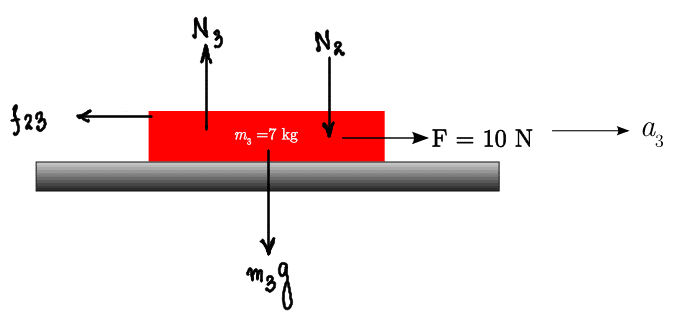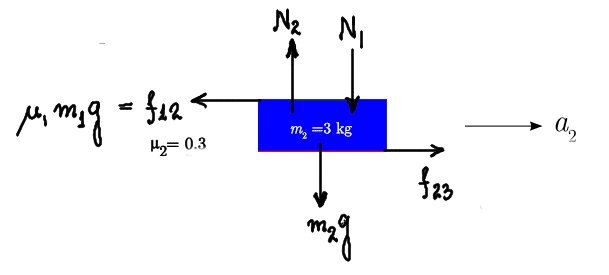# External force on three blocks stacked

brotherbobby
Homework Statement:
An external force of 10 N is applied on the lowest of the three blocks as shown in the figure below. The masses of the blocks are shown along with the coefficient of friction of their (rough) surfaces. If the ground in contact with the lowest block is smooth, calculate the accelerations ##a_1, a_2\; \text{and}\; a_3## of the three blocks. [Assume ##g = 10 m/s^2##]
Relevant Equations:
Newton's (2nd) law : ##\Sigma \vec F = m\vec a##. Frictional force ##f =\mu N##, where ##N## is the normal reaction at contact and ##\mu## is the coefficient of friction.The figure is shown above. In order to facilitate solution, I need to separate each block and draw the free-body diagram (FBD) with all the forces acting on it.

1. Let me start with the lowest block. Let me put its FBD as shown below.For forces in the vertical direction, ##N_3 = N_2 + m_3 g = (m_1+m_2)g + m_3g = (m_1+m_2+m_3)g##. The frictional force ##f_{23} = \mu_2 N_2 = \mu_2 (m_1+m_2)g##.
The net force acting on the block : ##F - \mu_2 (m_1+m_2)g = 10 - 0.3(2+3) \times 10 = 10-15 = -5## N, which is less than zero.

Hence the lowest block will not move relative to the 2nd block above it (##\checkmark##, correct in so far as it matches with the answer in the text, see below). The block may have an acceleration, but certainly none relative to the block (2) above it. Please note that this, in and of itself, does not tell us what the frictional force between the two blocks (##f_{23}##) is. It has to be of some value >0 but its value as of yet is unknown. What we can say is that ##F - f_{23} = m_3 a##.

2. I put the FBD of block 2. This is where my problems begin.Since we don't know ##f_{23}## (see 1 above), we don't know whether it is more or less than the maximum frictional force between the blocks 1 and 2 ##f_{12}##. I could not proceed further.

[The answer as given in the text is that the three blocks move as a system with acceleration ##a = \frac{F}{m_1+m_2+m+3} = \frac{10}{12} = \mathbf{\boxed{5/6 \; \text{ms}^{-2}}}##.]

In my attempt for the lowest block (1) above, I find that I did go somewhere. Indeed, far as I can see, blocks 2 and 3 won't move relative to one another. How does that mean that the system of blocks 2-3 won't move relative to block 1?

Some help as to how that is would be appreciated.

Last edited:

Homework Helper
Gold Member
Begin by calculating the acceleration of the blocks as if they did not slide relative to each other. Note that the net force on the top block under this assumption is the static friction ##f_{s1}##. Then answer the question, "Is the maximum value ##f_{s1}^{max}## greater than or less than ##m_1a##?" If less than, ##m_1## is sliding on ##m_2##; if greater than, it is not. Repeat considering ##m_1+m_2## as the "top" block.

•nasu
teo11
Begin by calculating the acceleration of the blocks as if they did not slide relative to each other. Note that the net force on the top block under this assumption is the static friction ##f_{s1}##. Then answer the question, "Is the maximum value ##f_{s1}^{max}## greater than or less than ##m_1a##?" If less than, ##m_1## is sliding on ##m_2##; if greater than, it is not. Repeat considering ##m_1+m_2## as the "top" block.
Sorry to bother but do you mean the acceleration of blocks as if m1 and m2 only didn’t slide relative to each other in first line because if I consider acceleration such that all three blocks don’t slide relative to each other and then compare the max frictional value, answer is coming wrong in some cases.
Thanks

Homework Helper
Gold Member
2022 Award
Sorry to bother but do you mean the acceleration of blocks as if m1 and m2 only didn’t slide relative to each other in first line because if I consider acceleration such that all three blocks don’t slide relative to each other and then compare the max frictional value, answer is coming wrong in some cases.•